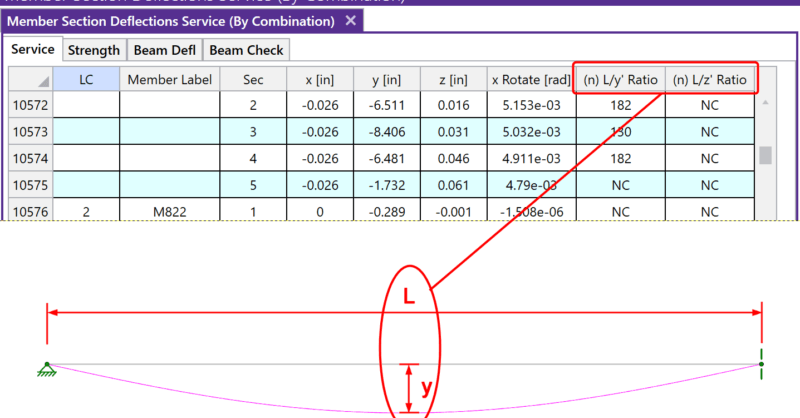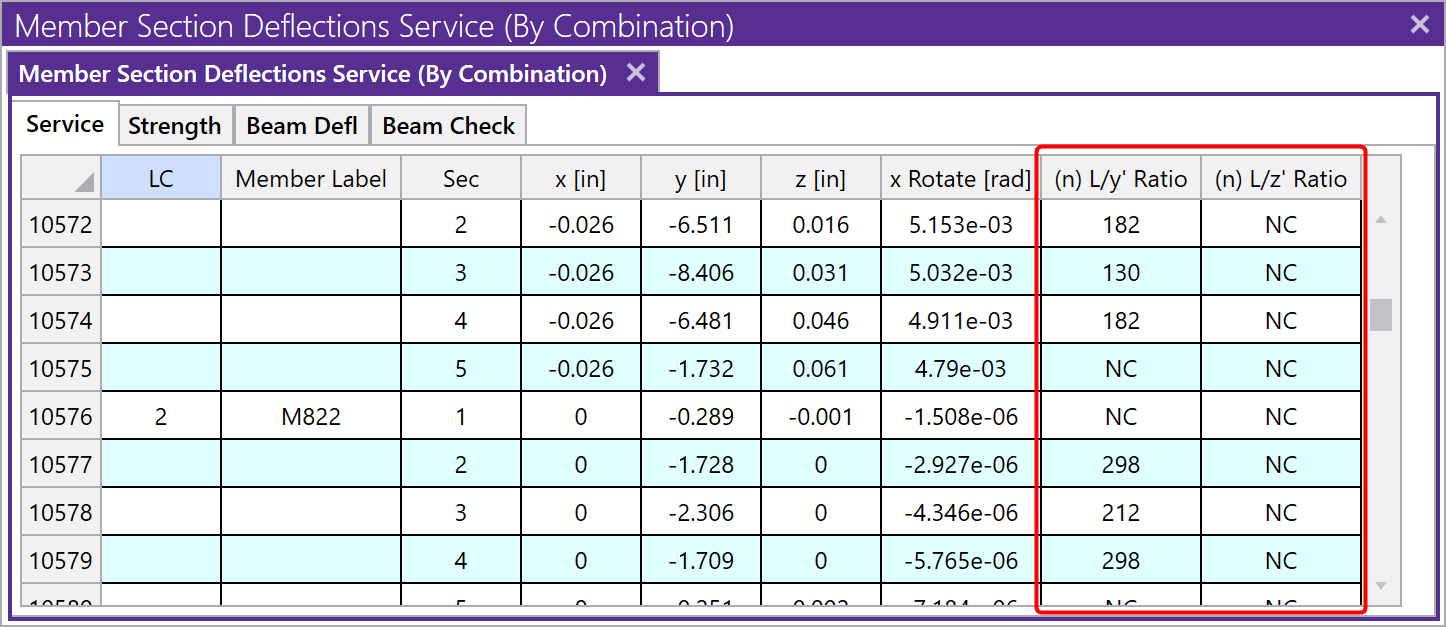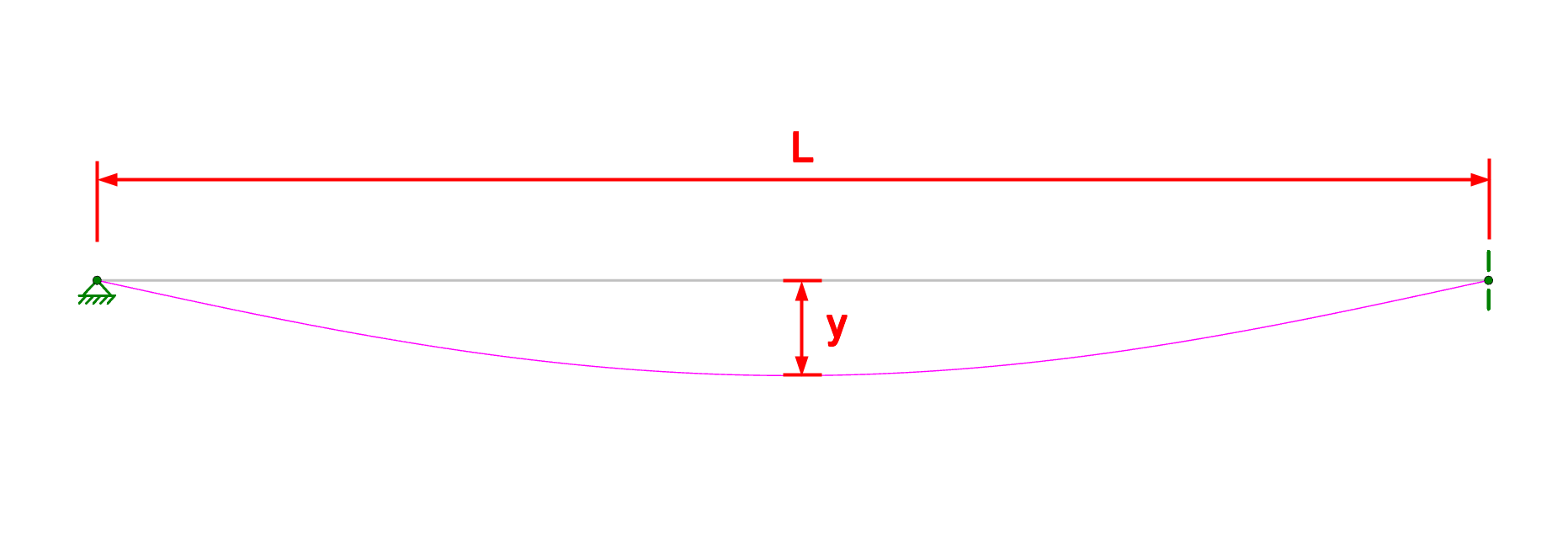# How RISA-3D Calculates Member Deflection Ratios

After solving a model, you will see in the Member Deflections spreadsheet the following deflection ratios:These ratios are calculated using the full length of the member (L) minus any member end offsets. This length does not consider individual span length in continuous span members. Also the length does not consider 2*L for the length of cantilevers. It is RISA’s plans to consider these features for a future enhancement of the program.

The variables y and z are the local y-axis and z-axis deflections relative to the straight line between the deflected positions of the end joints. For cantilevers the deflection is relative to the original position of the member.Expressed as an equation, n = L/deflection, where n is what is tabulated in the spreadsheet above. The smaller the deflection, the larger the n value will be. If ‘NC’ is listed, that means the ‘n’ value is greater than 10000 which is a very small deflection. The minimum value that will be shown is ‘1’. For example, if the deflection criteria is L/360, check here to make sure no tabulated values are less than 360. Greater than 360 is sufficient.